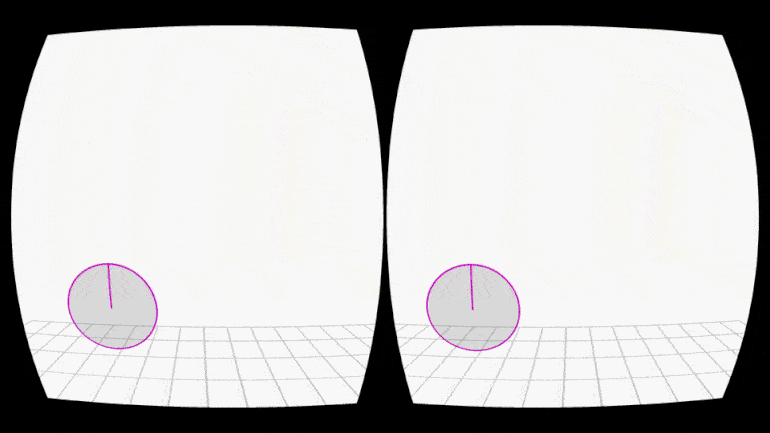payod.panda@gmail.com

# VR Function Generator

An intuitive three dimensional mathematical function generator in Virtual Reality (VR).

January - February, 2016

During my graduate research on helping designers understand code, I realized that a lot of designers are intimidated by mathematical functions. This project was an exploration in how to make the process of thinking about mathematical functions more intuitive for visual thinkers.

During a conversation with one of my colleagues about a piece of code he was having trouble writing, he described the working behind what he wanted the code to do perfectly. In doing so, he also described the mathematical functions controlling a certain variable in the program by way of moving his hands in the air — creating the perceived shape of the mathematical function in thin air — without knowing what the mathematical expression for that function was.

Further exploration and inquiry as part of my research informed me that one of the primary reasons for being intimidated by and a barrier to learning math came from the presentation — long winded expressions, lots of variables, lots of numbers. What if there were a more visual, intuitive way of expressing a function?

I designed this function generator fueled by how my friend and colleague had defined the mathematical function by waving his hands and being inspired by graphical representations of mathematical functions. I decided to implement this in VR because I wanted it to be as natural as possible for designers. We are used to explaining things by expressing, we express by drawing, by moving our hands. With my implementation the mode of input is the user's hands, rather than a controller or a pointing device like a mouse.

I make use of the Leap Motion controller in tandem with a VR headset (I used the Oculus Rift).The interface is a gestural interface, where the user can draw three dimensional shapes by moving his or her hands. These shapes express the function generated for the value of a dependent variable (say, distance moved, f(x)) wrt the independent variable (say, time, x).My implementation makes use of an extrapolation algorithm. The shape defined by the user acts as a seed for the generated function, and the algorithm tries to extrapolate it further. The user can turn the extrapolation view on or off, and decide the level of extrapolation. Currently the algorithm assumes a periodic nature and takes into account the general trend, to make sure that the generated function is continuous.
The extrapolation has a different opacity so it's easy for the user to distinguish between the function and its extrapolation.From a user experience perspective, the extrapolation introduces an element of play into the process. Increasing the levels of extrapolation to a large number, it can be very gratifying to see a generated shape being extrapolated to near-infinity in VR. :)